# Figuring out the potential energy

PhyIsOhSoHard

## Homework Statement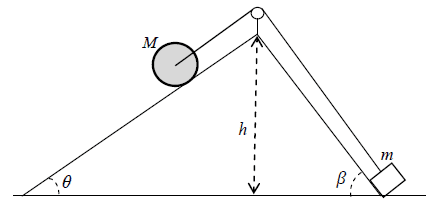A cylinder with mass M and radius R and moment of inertia I is standing on a rough surface (the left part of the triangle).
A box with mass m is standing on a smooth surface (right part of the triangle).
The cylinger and box is connected by a massless rope over a massless pulley.

The cylinder starts from rest and then rolls. Find the velocity of the box at the top of the triangle.

## Homework Equations

Kinetic rotational and translational energy
Potential energy

## The Attempt at a Solution

I tried to solve this and then I looked at my facits but there was one thing I did not take account for in my energy equation.

This is my facit (point of reference for potential energy is at their start position):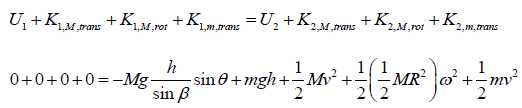Where "1" is their start positions and "2" is when the box reaches the top of the triangle.

The thing that I have problem figuring out is this part: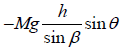I know it's supposed to be the potential energy of the cylinder when the box reaches the top, but I have no idea how they reached that. I've been looking at the drawing for an hour and can't figure it out.

Homework Helper
Gold Member
2022 Award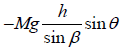I know it's supposed to be the potential energy of the cylinder when the box reaches the top, but I have no idea how they reached that. I've been looking at the drawing for an hour and can't figure it out.
Put in an unknown, d say, for the distance the mass will have moved. How far will the roller have moved? Given that the roller has moved that far down the slope of angle theta, how far has it moved vertically? How does d relate to h and beta?

•PhyIsOhSoHard
PhyIsOhSoHard
Put in an unknown, d say, for the distance the mass will have moved. How far will the roller have moved? Given that the roller has moved that far down the slope of angle theta, how far has it moved vertically? How does d relate to h and beta?
Alright let me see...

I drew this line in the drawing: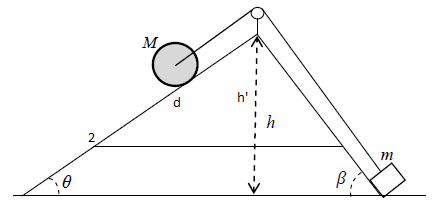The number "2" on the left indicates the final position where the cylinder is when the box is at the top.
If we assume that "d" is the distance down the triangle that the cylinder rolls and if h' is the height of the potential energy for the cylinder that we're looking for, then using the newly created triangle we get:
h' = d * sin(theta)

But now I just need an expression for "d". But now I'm again stuck. I seem to have trouble trying to relate d to beta.

Homework Helper
Gold Member
2022 Award
If we assume that "d" is the distance down the triangle that the cylinder rolls
I defined d as the distance the mass moves up. Is that the distance the roller moves down?
If it is, write another d where the mass slides up, and look at how that relates to h and beta.

•PhyIsOhSoHard
PhyIsOhSoHard
I defined d as the distance the mass moves up. Is that the distance the roller moves down?
If it is, write another d where the mass slides up, and look at how that relates to h and beta.

Ahh, I mixed them up. Ok I changed the drawing so now d is the distance the mass moves up and x is the distance the roller moves down: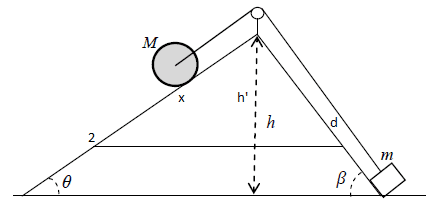So I have:
h' = x * sin(theta)

And:
h = d * sin(beta)

And... I know that the distance that both the mass and roller travels are equal to each other:
x = d
Thus:
h' = h/sin(beta) * sin(theta)

So that's how they came up with that expression. :)
However, I'm a bit confused at the negative sign in front of the expression. Are you supposed to add a negative sign because the roller moves in the opposite direction from the mass? (the roller moves down h whereas the mass moves up h)

•